# Island Rotation Frequency

Equations (11.144) and (12.3) yield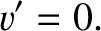(12.4)

It follows from Equation (11.136) that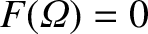. Hence, Equations (11.113) and (11.130) imply that the MHD stream-function of an isolated magnetic island chain takes the form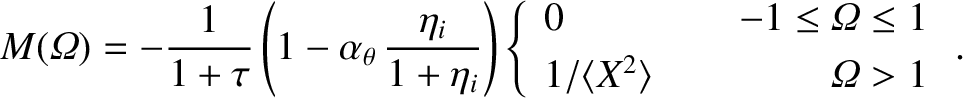(12.5)

Here,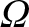is a magnetic flux-surface label that takes the value zero at the island O-points, unity on the magnetic separatrix, and is much larger that unity far from the island chain [see Equation (11.101)]. Moreover,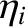is a dimensionless measure of the ion temperature gradient at the rational surface [see Equation (4.4)], and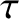the ratio of the electron and ion pressure gradients at the rational surface [see Equation (4.5)]. The quantity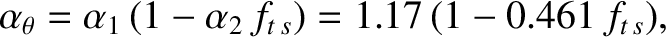(12.6)

where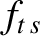is the fraction of trapped particles at the rational surface [see Equation (2.202)], parameterizes the influence of ion neoclassical poloidal flow-damping [see Equations (2.217), (2.218), and (11.5)]. Finally, the function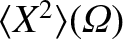is specified in Section 8.11. Note that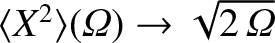as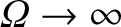. It follows from Equation (12.5) that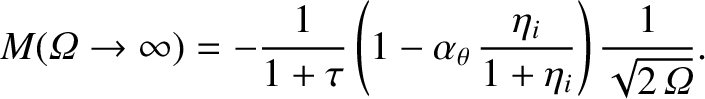(12.7)

However, Equations (11.109) and (12.4) yield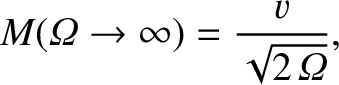(12.8)

where [see Equation (11.88)]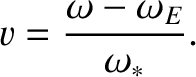(12.9)

Here,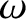is the rotation frequency of magnetic island chain in the laboratory frame (see Section 5.4),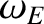the E-cross-B frequency at the rational surface [see Equation (5.44)], and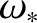the diamagnetic frequency at the rational surface [see Equation (5.47)]. The previous three equations give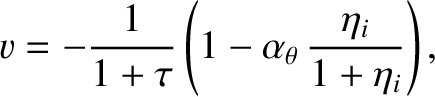(12.10)

which implies that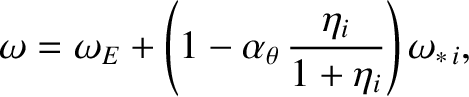(12.11)

where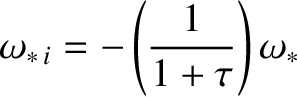(12.12)

is the ion diamagnetic frequency at the rational surface [see Equation (5.46)]. We conclude that, as a result of ion neoclassical poloidal flow-damping (i.e.,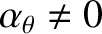), an isolated magnetic island chain does not exactly co-rotate with the unperturbed ion fluid at the rational surface (i.e.,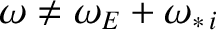). (Recall that non-neoclassical theory leads to the prediction that a wide magnetic island chain does exactly co-rotate with the unperturbed ion fluid at the rational surface. See Section 9.5.) Instead, assuming that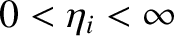, and given that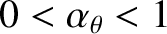under most circumstances [see Equation (12.6)], we deduce that a wide magnetic island chain propagates in the electron diamagnetic direction with respect to the unperturbed ion fluid at the rational surface, but in the ion diamagnetic direction with respect to the unperturbed MHD fluid [8,9,33]. This behavior should be contrasted with that of a linear tearing mode, which propagates in the electron diamagnetic direction with respect to the unperturbed MHD fluid. (See Chapter 6.)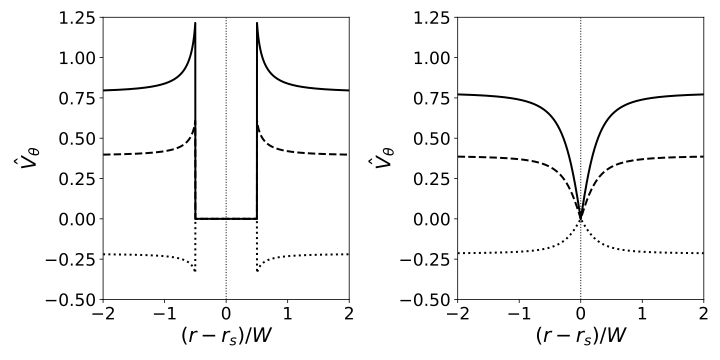According to Equations (8.75), (8.76), (11.130), and (12.5), the electron and ion fluid stream-functions take the respective forms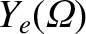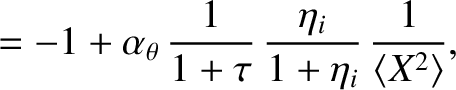(12.13)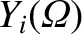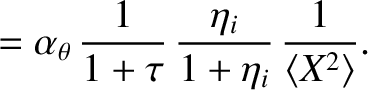(12.14)

The poloidal components of the perpendicular velocities of the electron, MHD, and ion fluids in the island rest frame are related to their respective stream-functions,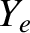,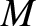, and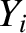, according to Equations (8.72)–(8.74).

Figure 12.1 shows typical normalized perpendicular velocity profiles of the electron, MHD, and ion fluids across the O-points and the X-points of an isolated magnetic island chain in the island rest frame, as determined from Equations (8.72)–(8.74), (12.5), (12.13), and (12.14). Here,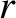is the magnetic flux-surface minor radius,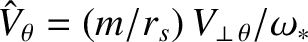, and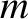is the poloidal mode number of the tearing mode. It can be seen that all three fluids co-rotate with the island chain inside the magnetic separatrix. However, unlike the non-neoclassical case (see Figure 9.3), ion neoclassical poloidal flow-damping (i.e.,) causes the ion fluid velocity profile to be modified by the presence of the island chain. In fact, all three velocity profiles are discontinuous across the magnetic separatrix. As was explained in Section 8.9, these discontinuities are resolved in a thin layer of thickness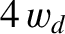on the separatrix. It is clear from the figure that the island chain rotates in the ion diamagnetic (i.e., negative) direction with respect to the unperturbed E-cross-B frame (i.e., the unperturbed MHD fluid) at the rational surface, but in the electron diamagnetic (i.e. positive) direction with respect to the unperturbed ion fluid.